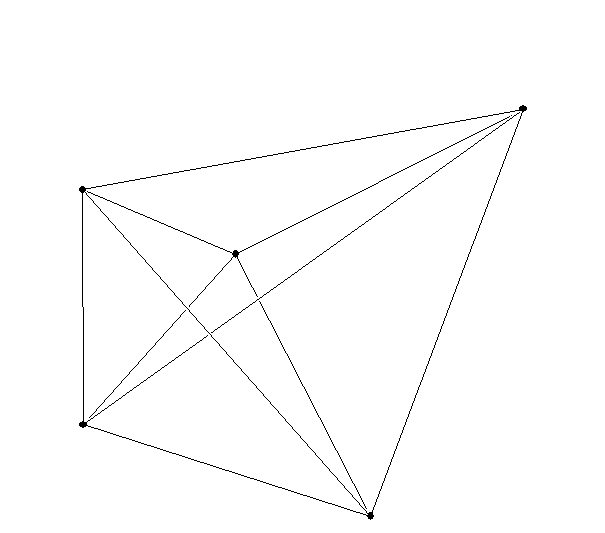April 9, 2014

The Tower of Hanoi "puzzle" Coding Activity

More thinking about a fourth (and higher) dimension and coding:
A progression: Point and segment on a line, line segment and square in a plane (2-dim), square and a cube in space (3-dim), cube and a "hypercube" in hyperspace (4-dim)

The  Hypercube and coordinates:
What do we measure?  How does this determine "dimension?"
For a Line segment we can use one number to indicate distance and direction from a single point:  0 .... 1

For a Square we use two "coordinates" and we can identify the vertices of the square: (0,0), (1,0), (0,1),(1,1)

For a Cube  we use three "coordinates" and we can identify the vertices of the cube with qualities such as "left..right", "up... down", and "front ... back":
(0,0,0) , (1,0,0), (0,1,0),(1,1,0)
(0,0,1), (1,0,1), (0,1,1), (1,1,1)

For a Hypercube....we use four "coordinates" and we can identify the vertices of the hypercube with qualities such as "left..right", "up... down", and "front ... back" and "inside... outside": (0,0,0,0) , (1,0,0,0), (0,1,0,0),(1,1,0,0)
(0,0,1,0), (1,0,1,0), (0,1,1,0), (1,1,1,0)
(0,0,0,1) , (1,0,0,1), (0,1,0,1),(1,1,0,1)
(0,0,1,1), (1,0,1,1), (0,1,1,1), (1,1,1,1)

Another four dimensional object:
The hyper simplex!point
line segment
triangle
tetrahedron ("simplex")

Cards and the fourth dimension.

(1,1,1,1)        (0,0,0,0)
(1,1,0,1)        (0,0,1,0)
(0,1,0,1)        (1,0,1,0)
(0,0,0,1)        (1,1,1,0)
(0,0,0,0)        (1,1,1,1)

Hamiltonian Tour:  move through each vertex once and only once.

Networks and maps on the Plane, the Sphere , and the Torus: See activity for networks on the torus.• Topology vocabulary:

"Bounded" In the plane- A line segment is bounded. A line is not bounded. A circle is bounded.  A parabola is not bounded. "Bounded" means the object can be visualized in a box.

"
closed
"  A line segment with endpoints is closed. A circle is closed.  A line is closed. A line segment missing one or both endpoints in not closed. A circle missing one point is not closed.

"
open
"  A line segment missing both endpoints is open. A line is open.
A circle missing one point is open.

"
connected
" A line, a line segment, a circle, a circle missing one point,  and a parabola are all connected.
A line segment missing an interior point is not connected.
It has two pieces.

The following two  letters   are topologically equivalent.

I   C

The following two  letters   are topologically equivalent to each other but not to the previous two letters. This can be seen by removing a single point from these letters which will not disconnect the curves, as it does with the previous letters.

O  D

The letter T missing the point where the top meets the vertical line segment is not connected. It has three pieces.

The letter Y missing the point where the top meets the vertical line segment also is not connected. It has three pieces.
T and Y are topologically  the same (equivalent)! this can be seen by bending the tops to the letters up or down and stretching and shrinking the lengths as well.

Questions: Is a triangle topologically related to another triangle?
Yes. Stretch the sides and you'll also transform the angles.

Is an circle topologically related to a line?  No.
The circle is bounded and still connected when you remove a point.
The line is not bounded and is disconnected when you remove a point.

Is a triangle topologically related to a square?a circle? yes.